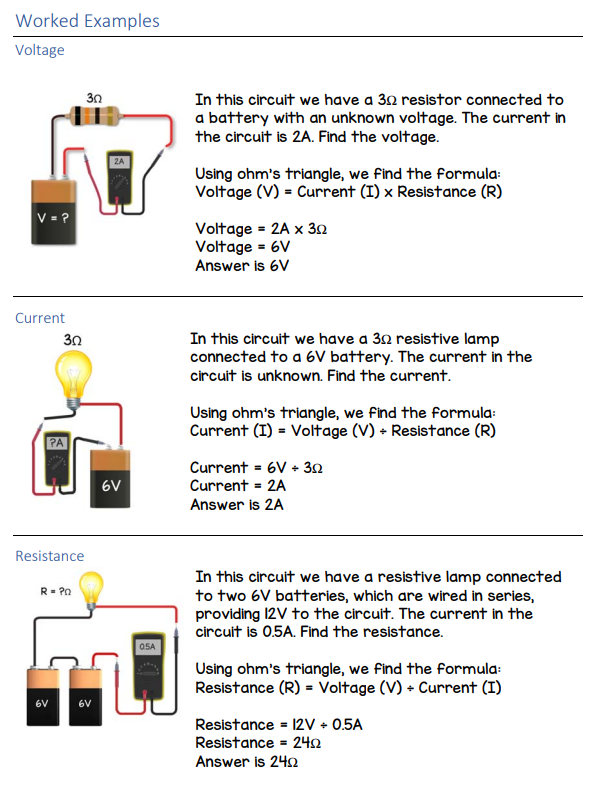# Current, Voltage, Resistance, Ohm's Law

## Current

The meaning of current in electronics is close to that of the current in a river.  Current is the amount of charge carried by electrons passing a point in a wire per second. The unit of current is the ampere, abbreviated as amp or as unit symbol A. For many circuits, one whole amp is quite a large current, so you will see the units of milliamp, mA.  1000mA = 1A## Voltage

The current will not flow without something influencing it. In a water pipe, that might be because one end of the pipe is higher than the other. To understand voltage, it can be useful to think of it as being similar to height in a system of water pipes. The common feature is that for voltage to make any sense, it must refer to two points. It is the difference in voltage that makes a current flow in a wire. If there is no difference in voltage between one end of a wire and another then no current will flow.  The unit of voltage is the volt, V.

## Resistance

Resistance is the ability of a substance to resist the flow of current. The thicker the wire, the less its resistance for a given length.

## Ohm's LawGeorg Ohm was a German physicist who discovered the law, named after him, which states that the current flow through a conductor is directly proportional to the potential difference (voltage) and inversely proportional to the resistance.